# sfepy.mechanics.shell10x module¶

Functions implementing the shell10x element.

Add additional strain components [Andelfinger and Ramm] (7 parameters to be condensed out).

sfepy.mechanics.shell10x.create_drl_transform(ebs)[source]

Create the transformation matrix for locking of the drilling rotations.

sfepy.mechanics.shell10x.create_elastic_tensor(young, poisson, shear_correction=True)[source]

Create the elastic tensor with the applied shear correction (the default) for the shell10x element.

sfepy.mechanics.shell10x.create_local_bases(coors)[source]

Create local orthonormal bases in each vertex of quadrilateral cells.

Parameters
coorsarray

The coordinates of cell vertices, shape (n_el, 4, 3).

Returns
ebsarray

The local bases, shape (n_el, 4, 3, 3). The basis vectors are rows of the (…, 3, 3) blocks.

sfepy.mechanics.shell10x.create_rotation_ops(ebs)[source]

Create operators associated to rotation DOFs.

Parameters
ebsarray

The local bases, shape (n_el, 4, 3, 3).

Returns
ropsarray

The rotation operators, shape (n_el, 4, 3, 3).

sfepy.mechanics.shell10x.create_strain_matrix(bfgm, dxidx, dsg)[source]

Create the strain operator matrix.

sfepy.mechanics.shell10x.create_strain_transform(mtx_ts)[source]

Create strain tensor transformation matrices, given coordinate transformation matrices.

Notes

Expresses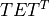in terms of symmetrix storage as, with the ordering of components:.

sfepy.mechanics.shell10x.create_transformation_matrix(coors)[source]

Create a transposed coordinate transformation matrix, that transforms 3D coordinates of quadrilateral cell vertices so that the transformed vertices of a plane cell are in theplane. The rotation is performed w.r.t. the centres of quadrilaterals.

Parameters
coorsarray

The coordinates of cell vertices, shape (n_el, 4, 3).

Returns
mtx_tarray

The transposed transformation matrix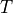, i.e..

Notes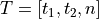, where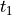,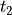, are unit in-plane (column) vectors andis the unit normal vector, all mutually orthonormal.

sfepy.mechanics.shell10x.get_dsg_strain(coors_loc, qp_coors)[source]

Compute DSG strain components.

Returns
dsgarray

The strain matrix components corresponding to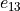,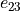, shape (n_el, n_qp, 2, 24).

Notes

Involves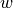,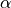,DOFs.

sfepy.mechanics.shell10x.get_mapping_data(ebs, rops, ps, coors_loc, qp_coors, qp_weights, special_dx3=False)[source]

Compute reference element mapping data for shell10x elements.

Notes

The code assumes that the quadrature points are w.r.t. (= thickness of the shell)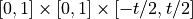reference cell and the quadrature weights are multiplied by.

sfepy.mechanics.shell10x.lock_drilling_rotations(mtx, ebs, coefs)[source]

Lock the drilling rotations in the stiffness matrix.

sfepy.mechanics.shell10x.rotate_elastic_tensor(mtx_d, bfu, ebs)[source]

Rotate the elastic tensor into the local coordinate system of each cell. The local coordinate system results from interpolation of ebs with the bilinear basis.

sfepy.mechanics.shell10x.transform_asm_matrices(out, mtx_t, blocks)[source]

Transform matrix assembling contributions to global coordinate system, one node at a time.

Parameters
outarray

The array of matrices, transformed in-place.

mtx_tarray

The array of transposed transformation matrices, see create_transformation_matrix().

blocksarray

The DOF blocks that are